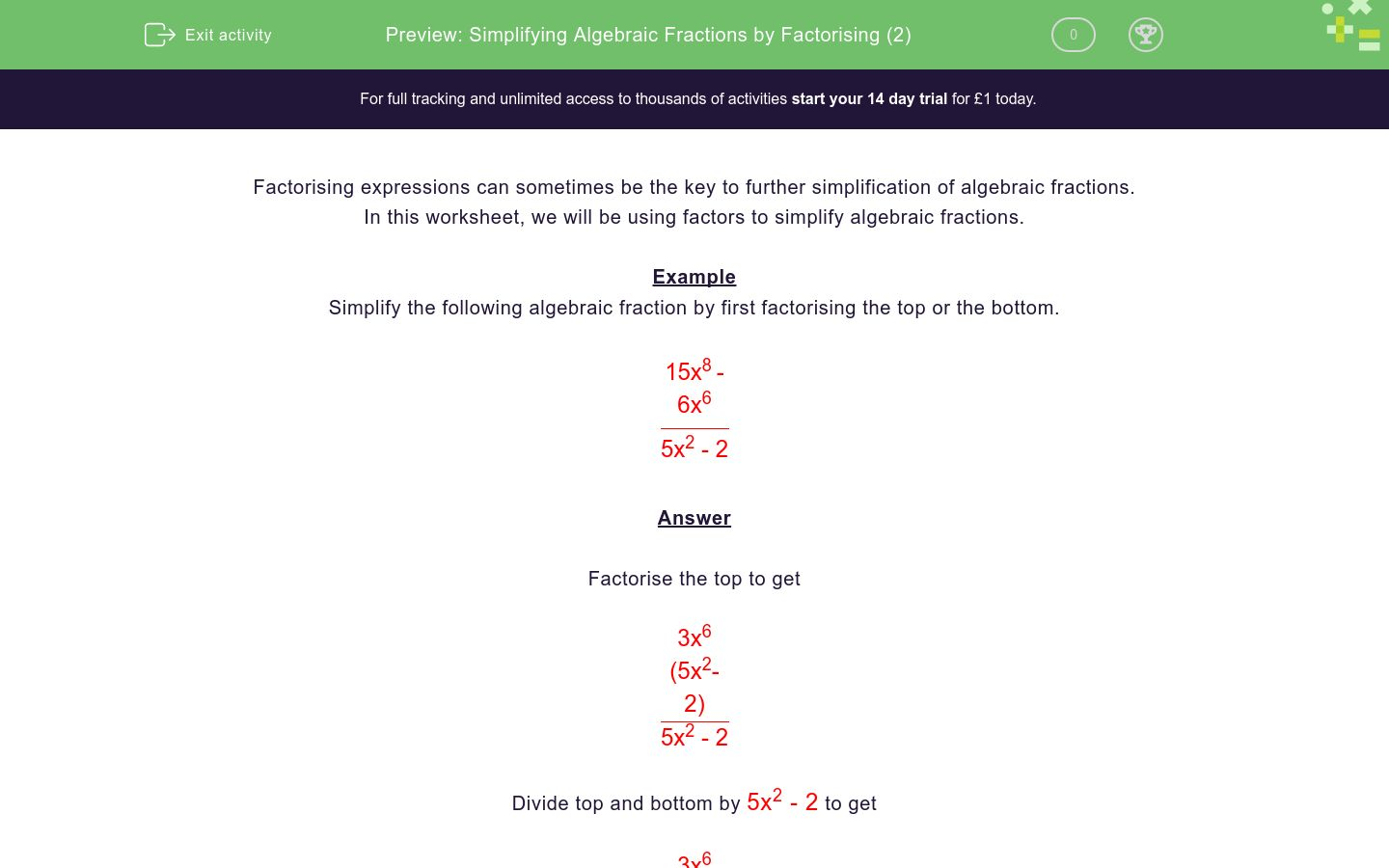# Simplifying Algebraic Fractions by Factorising (2)

In this worksheet, students simplify algebraic fractions by factorising.Key stage:  KS 4

Curriculum topic:  Algebra

Curriculum subtopic:  Simplify/Manipulate Algebraic Expressions/Fractions

Difficulty level:### QUESTION 1 of 10

Factorising expressions can sometimes be the key to further simplification of algebraic fractions.

In this worksheet, we will be using factors to simplify algebraic fractions.

Example

Simplify the following algebraic fraction by first factorising the top or the bottom.

 15x8 - 6x6 5x2 - 2

Factorise the top to get

 3x6 (5x2- 2) 5x2 - 2

Divide top and bottom by 5x2 - 2 to get

 3x6 (5x2- 2) 5x2 - 2

Simplify to get the answer

3x6

Simplify the following algebraic fraction by first factorising the top or the bottom.

 30x3 - 18x2 5x - 3

6x²

6x

x

Simplify the following algebraic fraction by first factorising the top or the bottom.

 18x4 - 18x3 3x - 3

6x³

6x

6x²

Simplify the following algebraic fraction by first factorising the top or the bottom.

 32x4 + 24x3 4x + 3

8x²

8x

8x³

Simplify the following algebraic fraction by first factorising the top or the bottom.

 28x4 + 21x2 4x2 + 3

7x³

7x²

7x

Simplify the following algebraic fraction by first factorising the top or the bottom.

 35x6 - 25x5 7x - 5

5x4

5x5

7x - 5

Simplify the following algebraic fraction by first factorising the top or the bottom.

 32x4 + 24x3 4x + 3

8x²

8x

8x³

Simplify the following algebraic fraction by first factorising the top or the bottom.

 30x4 - 18x2 5x2 - 3

6x²

6x

x

Simplify the following algebraic fraction by first factorising the top or the bottom.

 35x7 - 25x4 7x3 - 5

5x4

5x5

7x - 5

Simplify the following algebraic fraction by first factorising the top or the bottom.

 32x5 + 24x3 4x3 + 3x

8x²

8x

8x³

Simplify the following algebraic fraction by first factorising the top or the bottom.

 49x8 - 35x5 7x5 - 5x2

7x4

7x3

7x2

• Question 1

Simplify the following algebraic fraction by first factorising the top or the bottom.

 30x3 - 18x2 5x - 3

6x²
EDDIE SAYS
 6x2(5x - 3) 5x - 3
• Question 2

Simplify the following algebraic fraction by first factorising the top or the bottom.

 18x4 - 18x3 3x - 3

6x³
EDDIE SAYS
 6x3(3x - 3) 3x - 3
• Question 3

Simplify the following algebraic fraction by first factorising the top or the bottom.

 32x4 + 24x3 4x + 3

8x³
EDDIE SAYS
 8x3(4x + 3) 4x + 3
• Question 4

Simplify the following algebraic fraction by first factorising the top or the bottom.

 28x4 + 21x2 4x2 + 3

7x²
EDDIE SAYS
 7x2(4x2 + 3) 4x2 + 3
• Question 5

Simplify the following algebraic fraction by first factorising the top or the bottom.

 35x6 - 25x5 7x - 5

5x5
EDDIE SAYS
 5x5(7x - 5) 7x - 5
• Question 6

Simplify the following algebraic fraction by first factorising the top or the bottom.

 32x4 + 24x3 4x + 3

8x³
EDDIE SAYS
 8x3(4x + 3) 4x + 3
• Question 7

Simplify the following algebraic fraction by first factorising the top or the bottom.

 30x4 - 18x2 5x2 - 3

6x²
EDDIE SAYS
 6x2(5x2 - 3) 5x2 - 3
• Question 8

Simplify the following algebraic fraction by first factorising the top or the bottom.

 35x7 - 25x4 7x3 - 5

5x4
EDDIE SAYS
 5x4(7x3 - 5) 7x3 - 5
• Question 9

Simplify the following algebraic fraction by first factorising the top or the bottom.

 32x5 + 24x3 4x3 + 3x

8x²
EDDIE SAYS
 8x2(4x3 + 3x) 4x3 + 3x
• Question 10

Simplify the following algebraic fraction by first factorising the top or the bottom.

 49x8 - 35x5 7x5 - 5x2